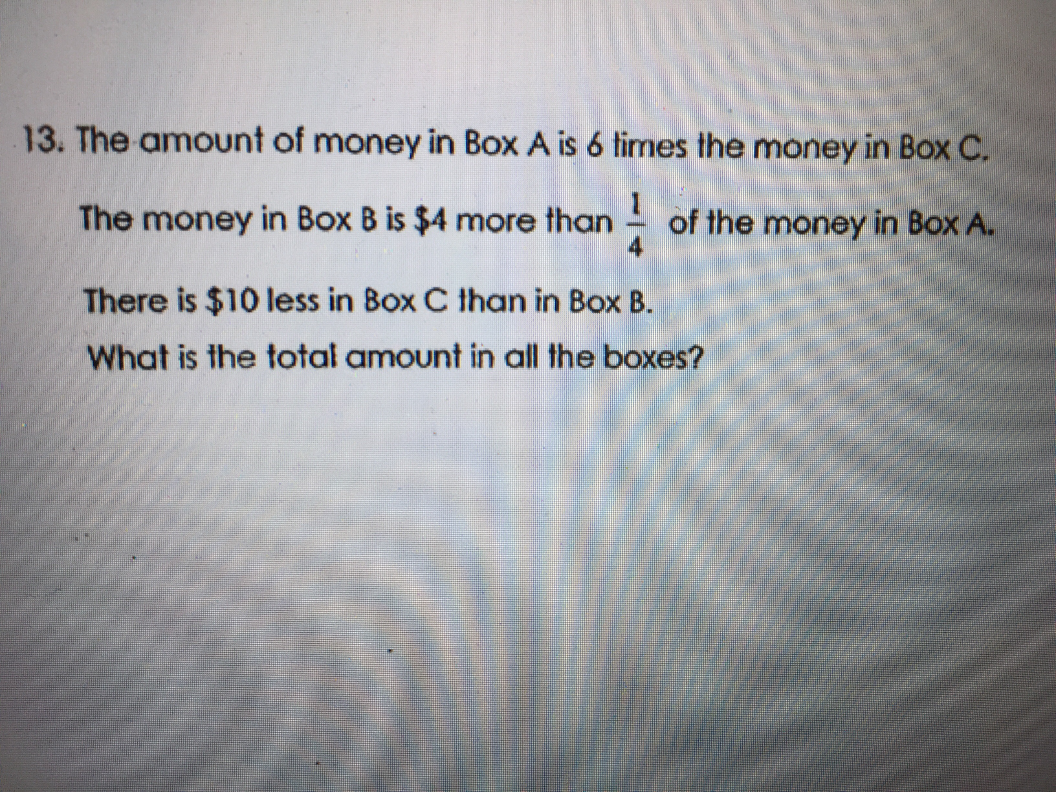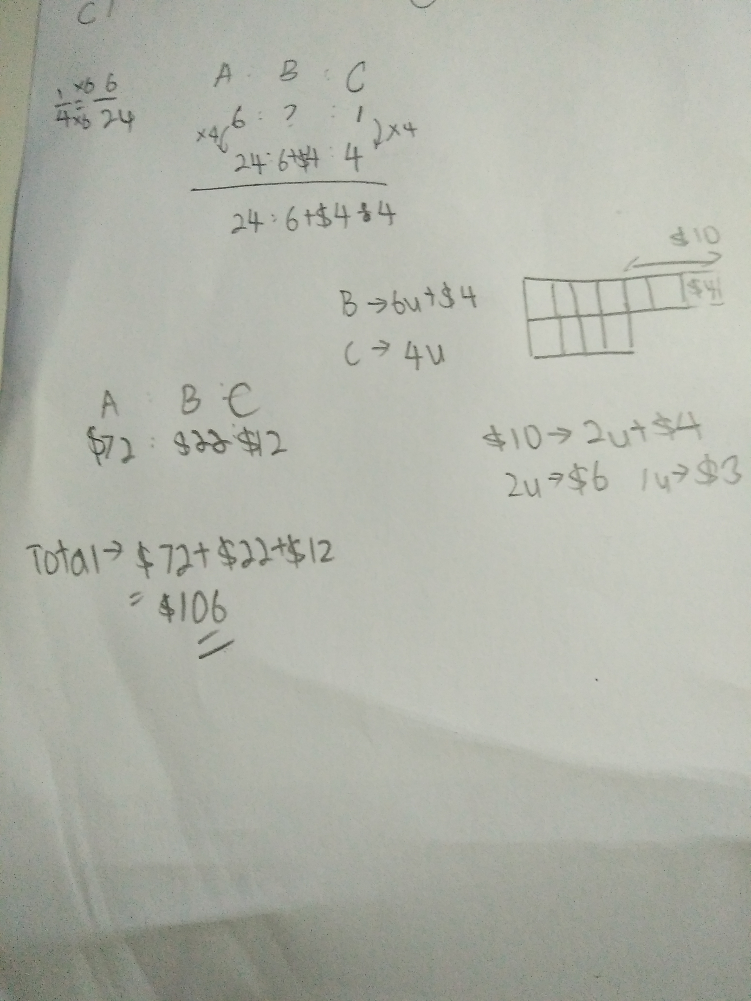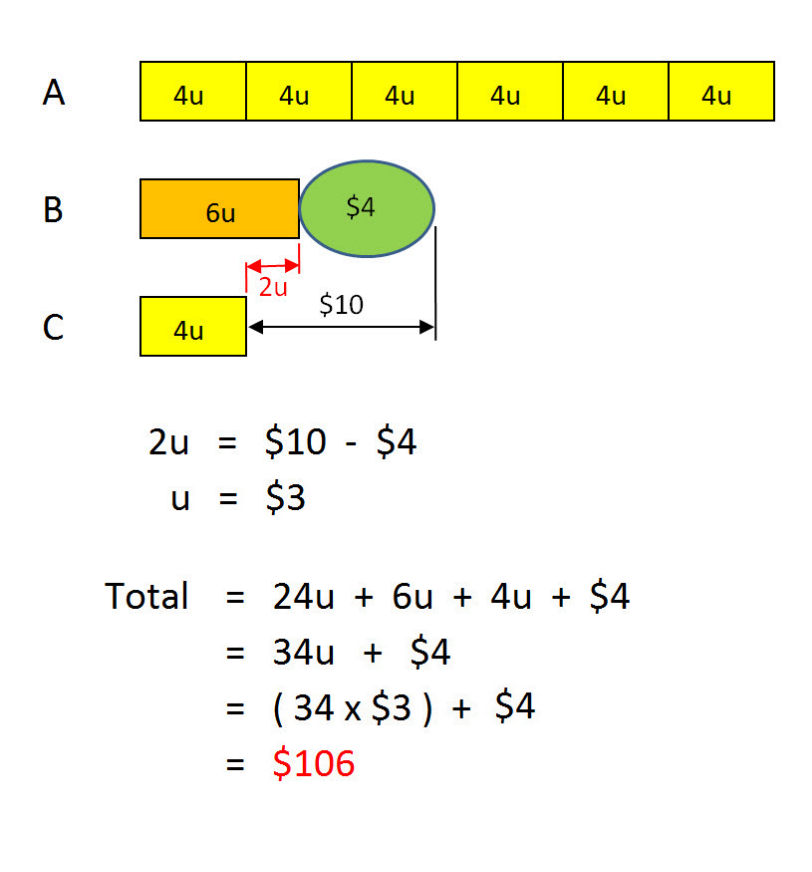# QuestionPls help me with this P5 question. Thks

Source: Assessment

Because “A” is initially 6 blocks and we need to make a quarter (1/4) out of this 6 blocks for “B”. So the easy way out is to subdivide each block of “A” into 4 smaller parts. So “A” becomes 24u total and then B becomes (24 x 1/4) = 6u. Hope this is clear.  😀

Thks! I understand now!

Welcome.  😀

0 Replies 0 Likes0 Replies 0 LikesSorry can explain why B is 6u and A each unit is 4u? Thks

2 Replies 0 Likes

Or you can also make “A” become total = (6×2) = 12u , and “B” become = (12u x 1/4) = 3u. The results will be the same.

0 Replies 1 Like

Because “A” is initially 6 blocks and we need to make a quarter (1/4) out of this 6 blocks for “B”. So the easy way out is to subdivide each block of “A” into 4 smaller parts. So “A” becomes 24u total and then B becomes (24 x 1/4) = 6u. Hope this is clear.  😀